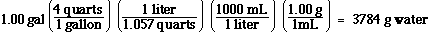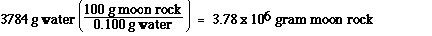### This is another density problem, because we are given a volume of water and told to determine the a mass. This problem is a little more complicated. We know how many grams of water are in 100. grams of moon rock. If the moon rock is 0.100% water by mass, then if we have 100 grams we know there is 0.1 grams of water. So how many grams of water are in a gallon of water is the next question. To determine that we'll begin with a gallon of water and convert that to grams of water in the following way. Oh, remember the density of water is 1.0 g ­ mL-1.### So now we know how many mLs there are in a gallon. We can now use density to find the grams,### we determine the mass of the moon rock which contains 3784 g of water.